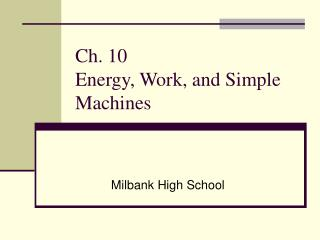Download PresentationCh. 10 Energy, Work, and Simple MachinesCh. 10 Energy, Work, and Simple Machines - PowerPoint PPT Presentation

Download PresentationCh. 10 Energy, Work, and Simple Machines
An Image/Link below is provided (as is) to download presentation

Download Policy: Content on the Website is provided to you AS IS for your information and personal use and may not be sold / licensed / shared on other websites without getting consent from its author. While downloading, if for some reason you are not able to download a presentation, the publisher may have deleted the file from their server.

- - - - - - - - - - - - - - - - - - - - - - - - - - - E N D - - - - - - - - - - - - - - - - - - - - - - - - - - -
Presentation Transcript

1. Ch. 10Energy, Work, and Simple Machines Milbank High School

2. Sec. 10.1 • Objectives • Describe the relationship between work and energy • Display an ability to calculate work done by a force • Identify the force that does work • Differentiate between work and power and correctly calculate power used

3. Energy • The ability to produce change in itself or the environment • Mechanical Energy • Ability to do work • Potential Energy • Stored energy • Kinetic Energy • Energy of motion

4. Kinetic Energy • Utilizes motion equations and Newton’s second law of motion • Produces

5. Work • Transfer of energy by means of forces • 1/2mv1 – 1/2mv0 = Fd so… • K = 1/2mv2 so… • W = Fd or… • K1 – K0 = W or ∆K = W • Measure in Joules (kg·m2/s2)

6. Problems • Example Problem Pg. 226 • Calculating Work Practice Problems Pg. 227

7. Calculating work at an angle • W = Fd cos Ө • Example Problem Pg. 228 • Force and Displacement at an Angle

8. Power • Power is the rate of doing work, or the rate at which energy is transferred • P = W/t • Power is measure in watts (1 joule of energy transferred in one second)

9. Sec. 10.2 • Obejctives • Demonstrate knowledge of why simple machines are useful • Communicate an understanding of mechanical advantage in ideal and real machines • Analyze compound machines and describe them in terms of simple machines • Calculate efficiencies for simple and compound machines

10. Machines • Ease the load by changing either the magnitude or the direction of a force as it trasmits energy to the task • Basically….make tasks easier • Wi • work input, the work you do • Wo • Work output, the work the machine does

11. Mechanical Advantage • The ratio of resistance force to effort force • Fr/Fe • Hopefully its greater than 1 • Effort force • The force you exert on a machine • Resistance force • Force exerted by the machine

12. Ideal Mechanical Advantage • Ideal Machine: all energy transferred, or in other words W0 = Wi Ideal Mechanical Advantage = de/dr Measure distances….with MA, you measure the forces

13. Efficiency • An ideal machine has equal output and input • Dpes it really ever happen? • Efficiency can be defined as W0/Wi x 100 (for a percent) • Or…MA/IMA x 100 • Efficient machines have an MA close to the IMA

14. Simple Machines • Lever • Pulley • Wheel and Axle • Inclined Plane • Wedge • Screw

15. Compound Machines • Consists of two or more simple machines • The resistance force of one becomes the effort force of the other • Example Problem Pg. 237 • Bicycle Wheel